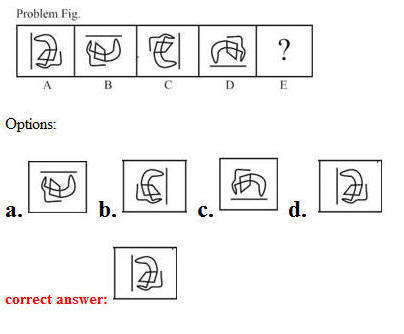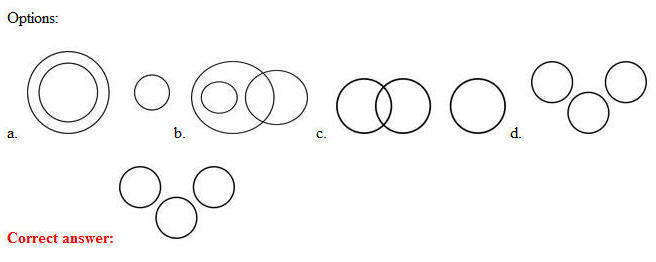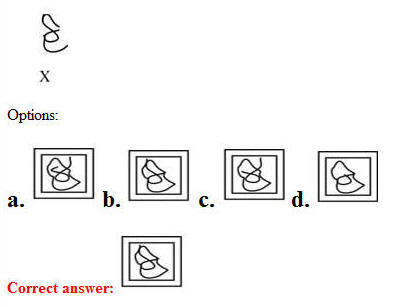# 11 July 2019 – Morning-Shift (Reasoning)

SSC CHSL (Tier – 1) Online Exam Paper – 2018 “held on 11 JULY 2019”

Morning-Shift (Reasoning)

Q1. Which letter-pair will replace the question mark (?) in the following series?
FJ, HL, ? , LP, NR

Options:

1. IN
2. GM
3. JM
4. JN

Q2. Select the option that is related to the third letter-pair in the same way as the second letter-pair is related to the first letter-pair.
DJ : HT : : HM : ?

Options:

1. LW
2. JK
3. LP
4. PY

Q3. Three of the following four letter-clusters are alike in a certain way and one is different. Pick the odd one out.

Options:

1. DFHJ
2. CEGI
3. QSUV
4. JLNP

Q4. A paper is folded and cut as shown in the following figure (). Select the option that depicts how this paper will appear when unfolded?Q5. Select the answer Fig. that will come next in the following Problem Fig. series.Q6. Three of the following four numbers are alike in a certain way and one is different.
Pick the number that is different from the rest.

Options:

1. 85
2. 65
3. 95
4. 75

Q7. Select the number-pair in which the two numbers are related in the same way as are the two numbers of the following number-pair.
29, 31

Options:

1. 23, 25
2. 15, 17
3. 11, 13
4. 20, 21

Q8. What was the day of the week on 15 August 2013?

Options:

1. Monday
2. Wednesday
3. Thursday
4. Tuesday

Q9. Which two signs should be interchanged in the following equation to make it correct?
5 + 8 – 30 × 10 ÷ 2 = 240

Options:

1. + and –
2. × and ÷
3. + and ÷
4. × and –

Q10. Select the correct mirror image of the given figure when the mirror is placed to the right of the figure.Q11. Select the Venn diagram that best illustrates the relationship between the following classes.
Rats, Frogs, SnakesQ12. Select the word-pair in which the two words are related in the same way as are the two words in the following word-pair.
Cement : Building

Options:

1. Pen : Pencil
2. Wire : Electricity
3. Book : Author
4. Wood : Furniture

Q13. How many triangle are present in the following figure?Options:

1. 10
2. 13
3. 9
4. 11

Q14. Three of the following four words are alike in a certain way and one is different.
Pick the odd word out.

Options:

1. Jeep
2. Ship
3. Car
4. Truck

Q15. Select the option in which the given figure (X) is embedded.Q16. In a code language, GOURD is written as IQSTF. How will APHID be written as in that language?

Options:

1. CREKF
2. CRJKF
3. CRFKF
4. CRFLF

Q17. Arrange the following words in the sequence as they appear in English dictionery order.

1. Mercury
2. Earth
3. Jupiter
4. Venus
5. Mars

Options:

1. 2, 3, 5, 1, 4
2. 4, 2, 5, 3, 1
3. 1, 4, 2, 3, 5
4. 2, 3, 1, 5, 4

Correct answer: 2, 3, 5, 1, 4

Q18. Select the set in which the numbers are related in the same way as are the numbers of the following set.
(49, 81, 121)

Options:

1. (16, 25, 49)
2. (16, 64, 100)
3. (36, 64, 100)
4. (9, 4, 8)

Q19. ‘ENGINEER’ is related to ‘BUILDING’ in the same way as ‘WRITER’ is related to ‘_________’.

Options:

1. BOOK
2. PEN
3. INK
4. PAPER

Q20. Select the set in which the numbers are related in the same way as are the numbers of the following set.
(16, 36, 64)

Options:

1. (12, 32, 50)
2. (10, 30, 58)
3. (6, 36, 80)
4. (9, 25, 62)

Q21. Anil and Abhay are brothers. Swati is the daughter of Samir and sister of Anil. How is Abhay’s mother related to Samir?

Options:

1. Sister-in-law
2. Sister
3. Mother
4. Wife

Q22. Which number will replace the question mark (?) in the following series?
2, 4, 7, 12, 19, 30, ?

Options:

1. 38
2. 47
3. 43
4. 36

Q23. If BARBER is coded as 116 and GLINT is coded as 73, then how will LIZARD be coded as?

Options:

1. 92
2. 91
3. 90
4. 93Q25. Three statements are given, followed by three conclusions numbered I, II and III. Assuming the statements to be true, even if they seem to be at variance with commonly known facts, decide which of the conclusions logically follow(s) from the statements.

Statements:

All hens are eggs.
All birds are eggs.
All eggs are lions.

Conclusions:

I. All hens are lions.
II. Some lions are eggs.
III. All birds are lions.

Options:

1. All the conclusions follow.
2. Only conclusion I follows.
3. Only conclusions I and II follow.
4. Only conclusions II and III follow.

Correct answer: All the conclusions follow.